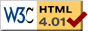### Journée-séminaire de combinatoire

#### (équipe CALIN du LIPN, université Paris-Nord, Villetaneuse)

Le 12 octobre 2021 à 14h00 en B107 (& visioconférence), Imre Bárány nous parlera de : Cells in the box and a hyperplane

Résumé : It is well known that a line can intersect at most $2n-1$ cells of the $n \times n$ chessboard. What happens in higher dimensions: how many cells of the $d$-dimensional $[0,n]^d$ box can a hyperplane intersect? We determine this number asymptotically. We also prove the integer analogue of the following fact. If $K,L$ are convex bodies in $R^d$ and $K \subset L$, then the surface area $K$ is smaller than that of $L$. Joint work with Peter Frankl.

[Slides.pdf] [arXiv]

 Dernière modification : Monday 24 January 2022Contact pour cette page : Cyril.Banderier at lipn.univ-paris13.fr